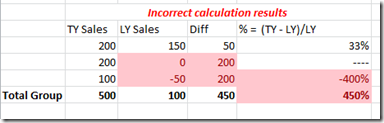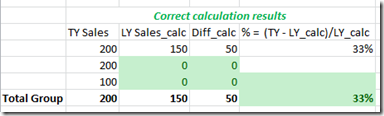## How to Filter out Last Year Value from a Percent Increase aggregation when the Last Year value is less than or equal to Zero

This is a sister solution to my last post which describes how to use math to ignore unwanted values in a cube query for an SSRS report, but still be able to aggregate the resulting values.  IIF statements can’t be aggregated, and aggregations can’t be nested, but math can always be aggregated in SSRS.

A common requirement is to calculate a Percent Increase over Last Year (LY).  The Percent Increase calculation is:

=(This Year – Last Year) / Last Year

But what if you want to ignore all values in a row where LY <= 0?  You can hide the detail rows using an IIF statement in your report, but to sum and calculate the percentage at a group level all values will be summed.  If this Year (TY) is more than zero it will be included in the % calculation, even though LY was less than or equal to zero.  And if LY is less than zero it will also be included in the sum.  This will skew the results and not give the desired results.  For example:The basic premise is that you want to zero out LY and TY in your calculation if LY <= 0.  Here’s how.

Add a calculated field to your Dataset Query called “LY_Inc_Multiple”.  Right click on the report Dataset, select Dataset Properties, Select Fields, click on the Add button and select Calculated Field.We want to create a calculation that will produce either a 1 if LY is positive or a 0 if LY is zero or negative.  We can then use this to multiply the values in the aggregate percent calculation for the Group to exclude any unwanted values from the result. Here I will divide LY by the absolute value of itself, plus one. The ‘plus one’ is to prevent division by zero if LY is zero. Take the Ceiling of this calculation will give you a result of either 1 or 0.

Click on the function button in the Field Source of your new LY_Inc_Multiple calculation.  Enter the following:

=(Ceiling(Fields!LY.Value/(ABS(Fields!LY.Value)+1)))

Here is how the calculation works, broken down step by step###### 3. USE YOUR CALCULATION IN THE REPORT

You can then go ahead and multiply your Increase Percent variables to zero out any unwanted values.  Be sure to use the LY_Inc_Multiple in your statement BEFORE summing.

For clarity, your calculation is this:

=(LY_Inc_Multiple*(TY-LY))/(LY_Inc_Multiple*LY)

In your report at the group level it manifests as this :

=Iif(Sum(Fields!LY_Inc_Multiple.Value*Fields!LY.Value)=0,"",((SUM(Fields!LY_Inc_Multiple.Value*(Fields!TY.Value-Fields!LY.Value))/Sum(Fields!LY_Inc_Multiple.Value*Fields!SunLY.Value))))

The IIF statement will hide any division by zero.

That’s basically it.  Each situation you come across will be slightly different, and you’ll need to think about the math you need for your particular situation.  It’s brain teaser for sure, but once you get the hang of it it can make report building much simpler.  You can avoid custom code solutions which have their own headaches, and you can control your summing and grouping without any funkiness.